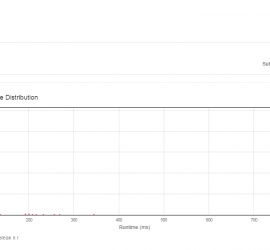# Daily Archives: August 8, 2015

## [leetcode] Insertion Sort List

Insertion Sort List Sort a linked list using insertion sort. Basic question. //Should I solve this problem in place? //Contains duplicates values? /** * Definition for singly-linked list. * struct ListNode { * int val; * ListNode *next; * ListNode(int x) : val(x), next(NULL) {} * }; */ class Solution […]## [leetcode] Binary Tree Postorder Traversal

Binary Tree Postorder Traversal Given a binary tree, return the postorder traversal of its nodes’ values. For example: Given binary tree {1,#,2,3}, 1 \ 2 / 3 return [3,2,1]. Note: Recursive solution is trivial, could you do it iteratively? Use two stacks to simulate recursion. stack st stores visiting nodes, […]## [leetcode] Reorder List

Reorder List Given a singly linked list L: L0→L1→…→Ln-1→Ln, reorder it to: L0→Ln→L1→Ln-1→L2→Ln-2→… You must do this in-place without altering the nodes’ values. For example, Given {1,2,3,4}, reorder it to {1,4,2,3}. The algorithm is straightforward. First, divide the linked list into two parts equally. (left part may be longer than […]## [leetcode] Word Break II

Word Break II Given a string s and a dictionary of words dict, add spaces in s to construct a sentence where each word is a valid dictionary word. Return all such possible sentences. For example, given s = “catsanddog”, dict = [“cat”, “cats”, “and”, “sand”, “dog”]. A solution is […]## [leetcode] Word Break

Word Break Given a string s and a dictionary of words dict, determine if s can be segmented into a space-separated sequence of one or more dictionary words. For example, given s = “leetcode”, dict = [“leet”, “code”]. Return true because “leetcode” can be segmented as “leet code”. First, I […]## [leetcode] Single Number II

Single Number II Given an array of integers, every element appears three times except for one. Find that single one. Note: Your algorithm should have a linear runtime complexity. Could you implement it without using extra memory? This is an extremely tricky problem. First, we consider each bit of the […]